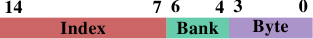# 6.2.1. 最佳化一階資料快取存取

$(AB)_{ij} = \sum^{N - 1}_{k = 0} a_{ik} b_{kj} = a_{i1} b_{1j} + a_{i2} b_{2j} + \cdots + a_{i(N - 1)} b_{(N - 1)j}$

for (i = 0; i < N; ++i)
for (j = 0; j < N; ++j)
for (k = 0; k < N; ++k)
res[i][j] += mul1[i][k] * mul2[k][j];


$(AB)_{ij} = \sum^{N - 1}_{k = 0} a_{ik} b^{\text{T}}_{jk} = a_{i1} b^{\text{T}}_{j1} + a_{i2} b^{\text{T}}_{j2} + \cdots + a_{i(N - 1)} b^{\text{T}}_{j(N - 1)}$

double tmp[N][N];
for (i = 0; i < N; ++i)
for (j = 0; j < N; ++j)
tmp[i][j] = mul2[j][i];
for (i = 0; i < N; ++i)
for (j = 0; j < N; ++j)
for (k = 0; k < N; ++k)
res[i][j] += mul1[i][k] * tmp[j][k];


sysconf (_SC_LEVEL1_DCACHE_LINESIZE)

gcc -DCLS=\$(getconf LEVEL1_DCACHE_LINESIZE) ...

#define SM (CLS / sizeof (double))
for (i = 0; i < N; i += SM)
for (j = 0; j < N; j += SM)
for (k = 0; k < N; k += SM)
for (i2 = 0, rres = &res[i][j],
rmul1 = &mul1[i][k]; i2 < SM;
++i2, rres += N, rmul1 += N)
for (k2 = 0, rmul2 = &mul2[k][j];
k2 < SM; ++k2, rmul2 += N)
for (j2 = 0; j2 < SM; ++j2)
rres[j2] += rmul1[k2] * rmul2[j2];


struct foo {
int a;
long fill;
int b;
};


pahole 輸出也能夠輕易看出元素是否必須被重新排列，以令那些一起用到的元素也會被儲存在一起。使用 pahole 工具，很容易就能夠確定哪些元素要在同個快取行，而不是必須在重新排列元素時才能達成。這並不是一個自動的過程，但這個工具能幫助很多。

1. 總是將最可能為關鍵字組的結構元素移到結構的開頭。
2. 存取資料結構、以及存取順序不受情況所約束時，以它們定義在結構中的順序來存取。

• 物件能夠以明確的對齊需求分配。對於動態分配（dynamic allocation），呼叫 malloc 僅會以相符於最嚴格的標準型別（通常是 long double）的對齊來分配物件。不過，使用 posix_memalign 請求較高的對齊也是可能的。

  #include <stdlib.h>
int posix_memalign(void **memptr,
size_t align,
size_t size);


這個函數將一個指到新分配的記憶體的指標儲存到由 memptr 指到的指標變數中。記憶體區塊大小為 size 位元組，並在 align 位元組邊界上對齊。

對於由編譯器分配的物件（在 .data.bss 等，以及在堆疊中），能夠使用一個變數屬性（attribute）：

  struct strtype variable
__attribute((aligned(64)));


在這個情況下，不管 strtype 結構的對齊需求為何，variable 都會在 64 位元組邊界上對齊。這對全域變數與自動變數也行得通。

對於陣列，這個方法並不如你可能預期的那般運作。只有陣列的第一個元素會被對齊，除非每個元素的大小是對齊值的倍數。這也代表了每個單一變數都必須被適當地標註。posix_memalign 的使用也不是完全不受控制的，因為對齊需求通常會導致碎片與／或更高的記憶體消耗。

• 一個使用者定義型別的對齊需求能夠使用一個型別屬性來改變：

  struct strtype {
...members...
} __attribute((aligned(64)));


這會使編譯器以合適的對齊來分配所有的物件，包含陣列。不過，程式設計師必須留意針對動態分配物件的合適對齊的請求。這裡必須再一次使用 posix_memalign。使用 gcc 提供的 alignof 運算子（operator）、並將這個值作為第二個參數傳遞給 posix_memalign 是很簡單的。

1. 產生的程式主動地對齊堆疊，必要時插入間隔。這需要程式檢查對齊、建立對齊、並在之後還原對齊。
2. 要求所有的呼叫端都將堆疊對齊。

-mpreferred-stack-boundary=2

struct order {
double price;
bool paid;
};


order 切成兩塊，前兩個欄位儲存在一個結構中，而另一個欄位儲存在別處要好得多了。這個改變無疑提高了程式的複雜度，但效能提升證明了這個成本的正當性。圖 6.6：AMD 上 L1d 的 Bank 位址

28. 我們這裡忽略了可能會改變上溢位（overflow）、下溢位（underflow）、或是四捨五入（rounding）的發生的算術影響。

29. 理論上在 1999 修訂版引入 C 語言的 restrict 關鍵字應該解決這個問題。不過編譯器還是不理解。原因主要是存在著太多不正確的程式碼，其會誤導編譯器、並導致它產生不正確的目的碼（object code）。
30. 測試是在一台 32 位元機器上執行的，因此 NPAD=15 代表每個串列元素一個 64 位元組快取行。